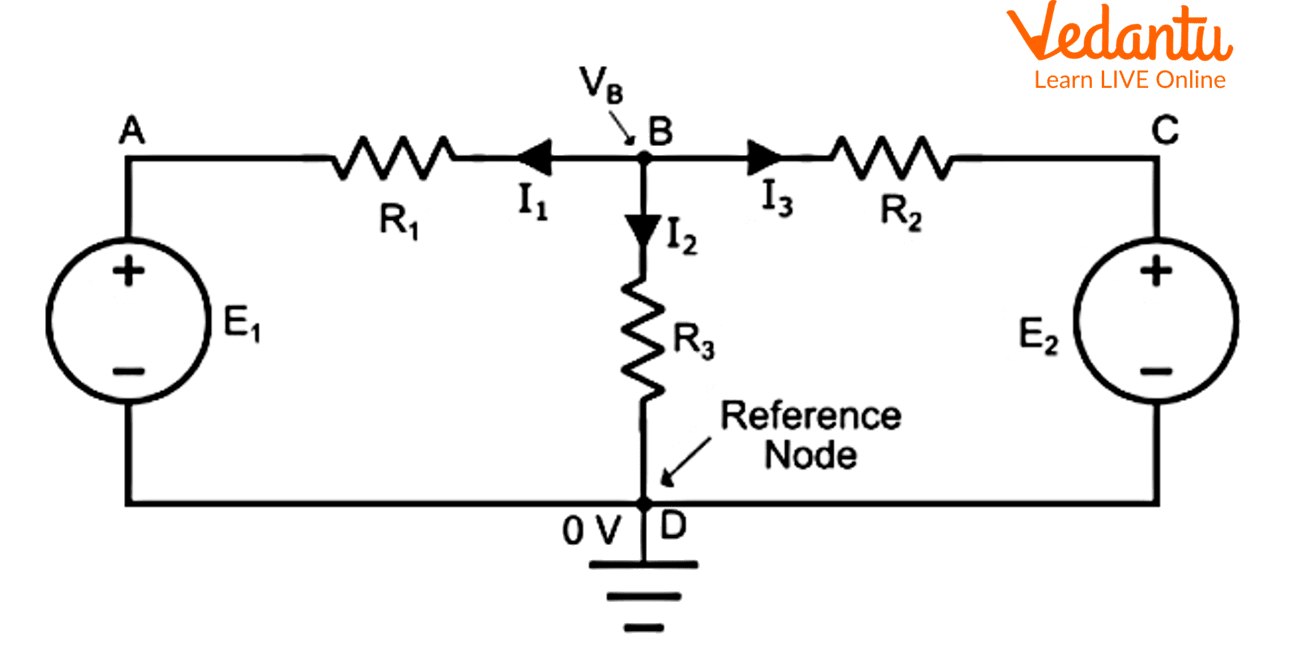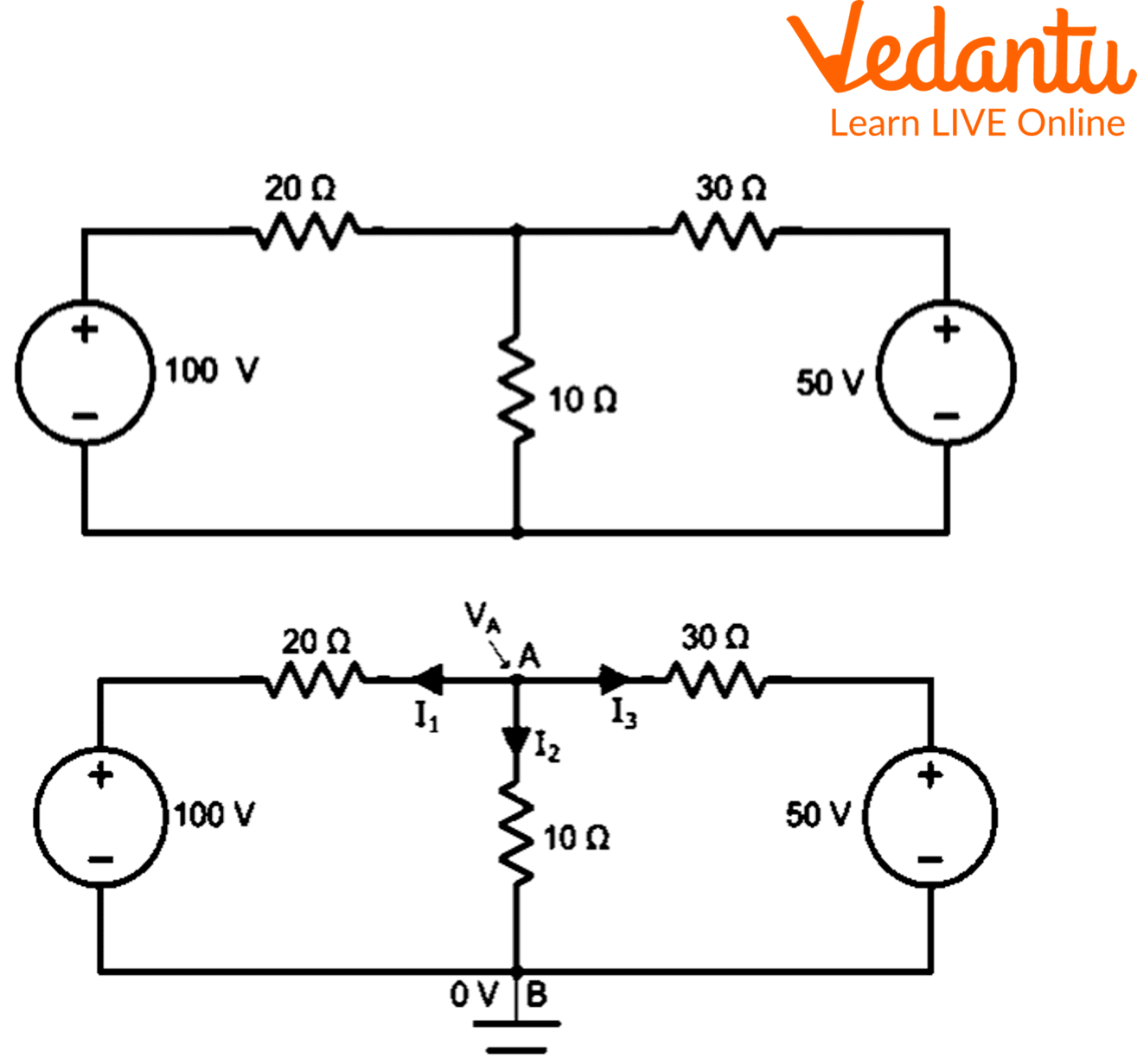Courses
Courses for Kids
Free study material
Free LIVE classes
More

# Nodal Analysis: A Detailed StudyLIVE
Join Vedantu’s FREE Mastercalss

## Nodal Analysis: An Introduction

Node voltages are used as the circuit variables in the nodal analysis, offering a general procedure for studying circuits. The Node-Voltage Method is an alternative name for nodal analysis. The characteristics of nodal analysis include:

• On the use of Kirchhoff's Current Law (KCL), nodal analysis is utilised

• There will be "n-1" simultaneous equations to solve with "n" nodes

• All of the node voltages can be found by solving 'n-1' equations

• The number of possible nodal equations is equal to the number of non-reference nodes.

Keep reading through the following content to learn more about nodal analysis, types of nodes, examples, solving circuits using nodal analysis and more.

## Kirchhoff’s Current Law

A technique for figuring out the branch currents in a circuit is termed nodal analysis. One of the nodes is chosen to serve as the reference node in this method. All the circuit's node potentials are calculated in relation to this reference node.

Kirchhoff's Current Law (KCL) asserts that "the algebraic sum of incoming currents and outgoing currents at a node is equal to zero," and serves as the foundation for the nodal analysis.

$\begin{array}{l}\sum {\mathop I\nolimits_{incoming} + } \mathop I\nolimits_{outgoing} = 0\\\end{array}$

• Node: In a network, a node is a location where two or more circuit elements converge.

• Junction: Junction is the place where three or more circuit elements come together. We must locate the potentials at the junction points rather than the nodes in the nodal analysis. There are fewer independent node-pair equations required than there are junctions in the network. In other words, if there are "n" independent node equations and "j" junctions, then:

$n = j - 1$

## Types of Nodes

• Non-Reference Node: It has a fixed node voltage.

• Reference Node: It serves as a point of reference for all other nodes in the network.

## Reference Node Types

• Chassis Ground: This kind of reference node serves as a common node for multiple circuits.

• Earth Ground: This type of reference node is known as Earth Ground when earth potential is utilised as a reference in any circuit.

## Step-by-step Procedures

To resolve nodal analysis in an electrical circuit or network, follow these nodal analysis steps below:

• Recognise the primary nodes in Step 1 and choose one of them to serve as the reference node. Thus, this node is handled the same as the ground.

• Mark all the primary node’s voltages with respect to the ground terminal in Step 2, excluding the reference node.

• Write nodal equations in Step 3 at each of the primary nodes aside from the reference node. You can arrive at this equation by first applying KCL and then Ohm's law.

• We must resolve the nodal equations we obtained in Step 3 to obtain the node voltages in Step 4.

## Difference Between Mesh and Nodal Analysis

In contrast to mesh analysis, which uses current values in a specific branch of a circuit, nodal analysis of an electrical network allows for the observation of voltages at a given branch. Mesh analysis is one type of technique used in the electrical network to solve planar circuits, specifically for the currents at any branch. Without putting any wires in contact with one another, these circuits can be sketched on a flat surface. Any circuit employs a more all-encompassing technique called loop analysis.

The KCL (Kirchhoff's Current Law) application is necessary for nodal analysis. The "n-1" instantaneous equations are required to solve n nodes. These equations can be solved to obtain all the node voltages. The number of acquirable nodal non-reference is equal to the number of reference nodes.

## Solving of Circuit Using Nodal Analysis

Look at the circuit below named Figure 1. A B, C, and D are the four nodes in the circuit, and nodes B and D are the junction points, with node D serving as the reference node.Nodal analysis problems for an explanation of the mathematical solution.

Hence, If node B has a higher voltage $\mathop V\nolimits_B$ than any of the other nodes in the circuit.

By putting KCL on node B, we get,

$\mathop I\nolimits_1 + \mathop I\nolimits_2 + \mathop I\nolimits_3 = 0............(1)$

The branch currents are now determined by Ohm's law to be,

$\mathop I\nolimits_1 = \dfrac{{\mathop V\nolimits_B - \mathop E\nolimits_1 - 0}}{{\mathop R\nolimits_1 }} = \dfrac{{\mathop V\nolimits_B - \mathop E\nolimits_1 }}{{\mathop R\nolimits_1 }}$

$\mathop I\nolimits_3 = \dfrac{{\mathop V\nolimits_B - \mathop E\nolimits_2 - 0}}{{\mathop R\nolimits_2 }} = \dfrac{{\mathop V\nolimits_B - \mathop E\nolimits_2 }}{{\mathop R\nolimits_2 }}$

${I_2} = \dfrac{V_B}{R_3}$

In equation (1), we obtain by substituting the values of $\mathop I\nolimits_1$, $\mathop I\nolimits_2$, and $\mathop I\nolimits_3$.

$\dfrac{{\mathop V\nolimits_B - \mathop E\nolimits_1 }}{{\mathop R\nolimits_1 }} + \dfrac{{\mathop V\nolimits_B - \mathop E\nolimits_2 }}{{\mathop R\nolimits_2 }} + \dfrac{{\mathop V\nolimits_B }}{{\mathop R\nolimits_3 }} = 0...........(2)$

The equation can be solved to determine the voltage $\mathop V\nolimits_B$ at node B. (2). Since the resistance values, $\mathop E\nolimits_1$, and $\mathop E\nolimits_2$ values are known. As a result, we can calculate the branch current values.

## Nodal Analysis Examples

By using nodal analysis, determine the currents in the various circuit branches shown in the accompanying Figure 2.Nodal Analysis Examples

As illustrated in the circuit schematic, indicate the currents in the various branches. The true direction of the current is the opposite of the assumed direction if the value of any current in the solution turns out to be negative. As the reference node, use point B. Thus,

$\mathop I\nolimits_1 + \mathop I\nolimits_2 + \mathop I\nolimits_3 = 0............(1)$

Using Ohm’s law,

$\mathop I\nolimits_1 = \dfrac{{\mathop V\nolimits_A - 100}}{{20}}$

$\mathop I\nolimits_2 = \dfrac{{\mathop V\nolimits_A }}{{10}}$

And

$\mathop I\nolimits_3 = \dfrac{{\mathop V\nolimits_A - 50}}{{30}}$

$\dfrac{{\mathop V\nolimits_A - 100}}{{20}} + \dfrac{{\mathop V\nolimits_A - 50}}{{30}} + \dfrac{{\mathop V\nolimits_A }}{{10}} = 0$

$\dfrac{{3(\mathop V\nolimits_A - 100)}}{{60}} + \dfrac{{2(\mathop V\nolimits_A - 50)}}{{60}} + \dfrac{{6\mathop V\nolimits_A }}{{60}} = 0$

$\mathop {3V}\nolimits_A - 300 + \mathop {6V}\nolimits_A + \mathop {2V}\nolimits_A - 100 = 0$

$\mathop {11V}\nolimits_A = 400$

$\mathop V\nolimits_A = \dfrac{{400}}{{11}} = 36.36V$

Current $\mathop I\nolimits_1 = \dfrac{{\mathop V\nolimits_A - 100}}{{20}} = \dfrac{{36.36 - 100}}{{20}} = - 3.182V$

Current $\mathop I\nolimits_2 = \dfrac{{\mathop V\nolimits_A }}{{20}} = \dfrac{{36.36}}{{20}} = 3.636V$

Current $\mathop I\nolimits_3 = \dfrac{{\mathop V\nolimits_A - 50}}{{30}} = \dfrac{{36.36 - 50}}{{30}} = - 0.455V$

The fact that currents have a negative sign indicates that their real directions are different from what was previously supposed.

## Conclusion

This was all about a general overview of nodal analysis. When the circuit diagram doesn't show any conductor lane crossings, nodal analysis can be applied for circuit analysis prominently and uses Kirchhoff's Current Law & node equations to solve voltage values within the circuit. When the voltage at the ground terminal is equal to 0 volts, this nodal analysis can be used to determine the voltage at each node with respect to a reference node that is typically known as ground.

Last updated date: 25th Sep 2023
Total views: 97.5k
Views today: 2.97k

## FAQs on Nodal Analysis: A Detailed Study

1. How many equations are there in a circuit with 12 nodes?

The number of equations we can obtain will always be smaller than the specified number of nodes because we are aware that one node is always regarded as a reference node. There are 11 equations that may be obtained because of this.

2. How does nodal analysis determine node voltage?

With nodal analysis, anyone can determine the voltage at each node of the given circuit. Assume that node 2 serves as the reference node, with zero voltage.

3. What is the Kirchhoff current law?

Kirchhoff's current law states that the current entering a node should be equal to the current leaving it.